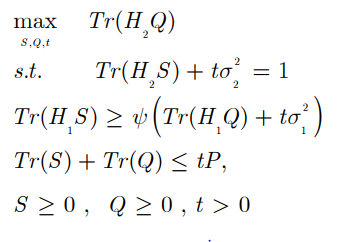# Infeasible Status

Hello @all !

I hope everyone is doing well.

I have convert my optimization problem into a suitable form so that Cvx can solve it, but still the status shows infeasible, anyone have idea about this?clear,clc %Clear all
m=2; %number of Tx and Rx antennas
n=1;
P=1; %Transmitted Power
%rng(1);
%H=rand(m,m); %Channel matrix
G1=rand(n,m);
G2=rand(n,m);
W1=rand(m,n);
W2=rand(m,n);
T1=W1W1’;
%t=0.5;
t=1/(trace(G2
G2’W1W1’)+1);
%Arho = [0.3 0; 0 0.3]; %Fix Arho
I=eye(m,m) %Identity matrix

%Arhobar=I-Arho %Arhobar

cvx_clear %To clear models used before
cvx_begin %To begin a new CVX model

variable T1(m,m) Symmetric %S Variable
maximize (trace(G2G2’W2W2’)); %Objective Function
subject to
(trace(G2
G2’*T1))+t==1;

trace(G1G1’T1)>=0.5(trace(G1G1’W2W2’)+t*I);

trace(T1)+trace(W2W2’)<= tP
t>0;
T1==semidefinite(m);
W2*W2’>=0;

cvx_end %To end the CVX model
cvx_status

The status is:

## Calling SDPT3 4.0: 6 variables, 2 equality constraints For improved efficiency, SDPT3 is solving the dual problem.

num. of constraints = 2
dim. of sdp var = 2, num. of sdp blk = 1
dim. of linear var = 3

SDPT3: Infeasible path-following algorithms

## number of iterations = 5 residual of dual infeasibility certificate X = 1.14e-27 reldist to infeas. <= 3.44e-28 Total CPU time (secs) = 0.18 CPU time per iteration = 0.04 termination code = 2 DIMACS: 2.8e-09 0.0e+00 8.1e-01 0.0e+00 -1.0e+00 2.0e-05

Status: Infeasible
Optimal value (cvx_optval): -Inf

cvx_status =

Infeasible

The trace constraints are not mutually compatible. Various combinations of trace constraints may be feasible, depending on the random input data you generated.

Do you have actual problem inputs, not just random inputs, to test this on?

1 Like

Dear Mark_L_Stone,

Actually, I don’t have the actual inputs because, I have two users and for two users I used two precoding matrices, that I have to optimize.

Feasibility may depend on the inputs. Perhaps “real” data results in a feasible problem, whereas random data generated per the probability distribution you are using, might (usually or always) not be

Anecdote: I recall Yinyu Ye once told me a random problem is either easy or infeasible. He was talking about LPs.

@Erling, I agree, because what matters is the multivariate probability distribution of the inputs. Real problems tend to have structure which is not manifested in independent uniform or Normal random numbers (everyone on this forum uses rand or randn to generate and populate independent random numbers in an entire matrix or vector).

You still might be able to achieve realistic structure by superimposing random numbers on an imposed structure or sparsity pattern, i.e., you only populate certain elements of a matrix with random numbers.

In fact I did just this on my final project for Gene Golub’s Statistical Computing course in March 1982. Using the for loop (one of the few programming features) and rand capabilities in the original Fortran-based MATLAB, I generated random matrices having specified structure or properties, such as (symmetric) psd (covariance matrices) , correlation matrices (psd matrices constrained to have unit diagonal), Toeplitz, circulant, Hurwitz and other special matrices. I systematically controlled the condition numbers to various specified levels. Then I examined the relation of condition number estimators in various norms to actual condition numbers in various norms. Over 24 years later, about a year and a half before he died,I talked to Golub at a conference. I mentioned that he gave me an A+ on that project. He asked me if I thought I deserved it. I said yes.Those were my last words ever to him. BTW, back in those more innocent (just pre-AIDS) days, long before important applications such as recommending which teen celebrities conservative old geezers should follow on social media, Golub referred in class to SVD as a Stanford social disease.

1 Like

Dear @Mark_L_Stone,

I have solved the problem, it gives me the simulation results sometimes and sometimes it shows the same -inf issue, i dont know what’s going wrong.

It sounds like your problem is feasible or not, depending on the input data. The :“solution” to your difficulties is to use real(istic) input data.If that is infeasible, then you need to examine hwo good your mode is.

1 Like